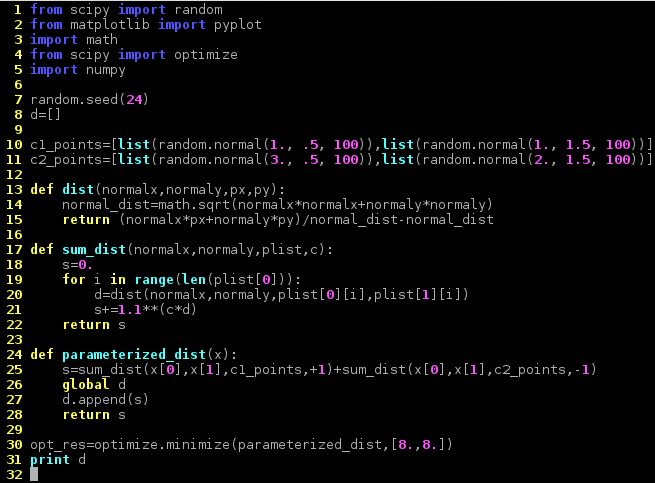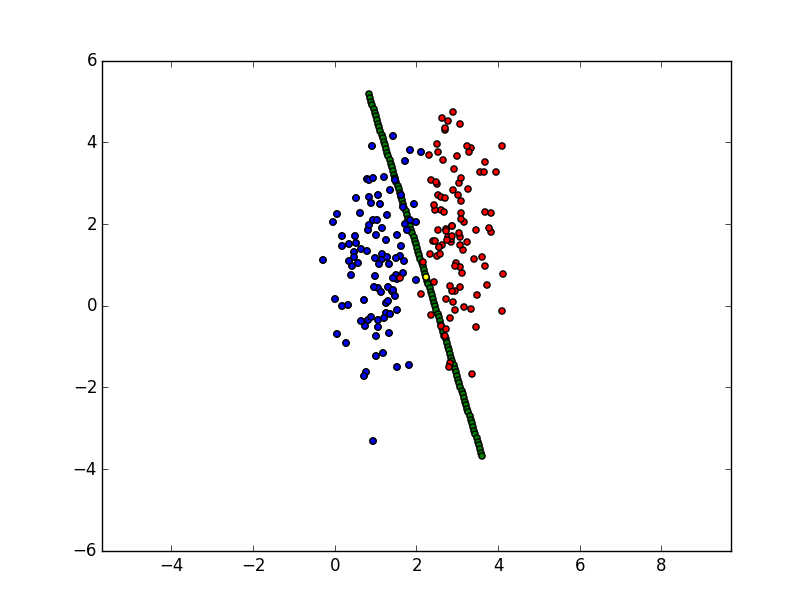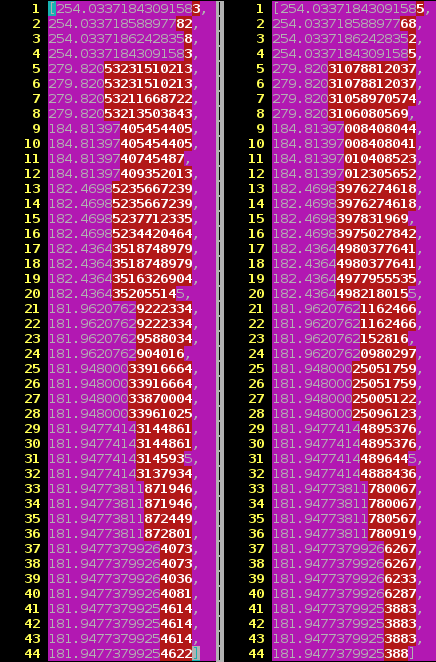# Implementation

In the following, the previously derived optimization problem has been implemented in a simple Python program.In the program two 2-dimensional Gaussian distributions are used to create sample points. The points are assigned two different labels identifying which distribution was used to create them. One distribution is centered around (1,1), the other is centered around (3,2).

These sample points are used in the training of the SVM to find the optimal plane that separates the two classes of points. Further points can now be classified to belong to either class depending on which side of the plane they reside on.

The following plot visualizes the two classes of points (blue and red) and the decision plane (here a line because we only deal with 2-dimensional data):# Parallelization

To enable this program to be executed on vast amounts of data the algorithm has to be parallelized. The following implementation in Python uses Apache Spark to achive this.Both programs output a quantity that reflects the distance of the plane to all points. The similarity of the output shows that the same problem is solved: